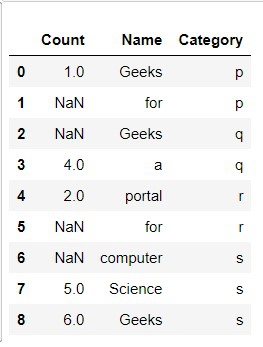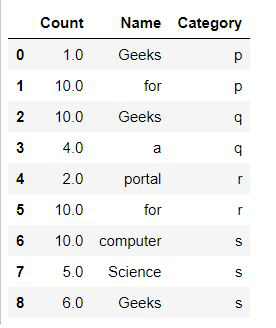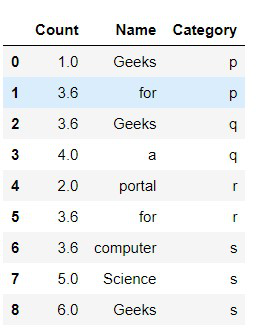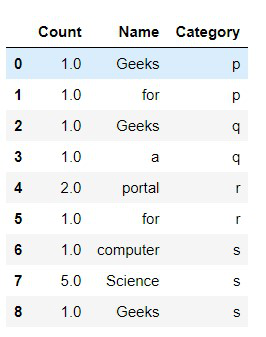# Fillna in multiple columns in place in Python Pandas

• Difficulty Level : Expert
• Last Updated : 07 Jul, 2021

In this article, we are going to write python script to fill multiple columns in place in Python using pandas library. A data frame is a 2D data structure that can be stored in CSV, Excel, .dB, SQL formats. We will be using Pandas Library of python to fill the missing values in Data Frame.

Let’s understand this with implementation:

Attention geek! Strengthen your foundations with the Python Programming Foundation Course and learn the basics.

To begin with, your interview preparations Enhance your Data Structures concepts with the Python DS Course. And to begin with your Machine Learning Journey, join the Machine Learning - Basic Level Course

First creating a Dataset with pandas

## Python3

 `# Importing Required Libraries``import` `pandas as pd``import` `numpy as np` `# Creating a sample dataframe with NaN values``dataframe ``=` `pd.DataFrame({``'Count'``: [``1``, np.nan,``                                    ``np.nan, ``4``,``                                    ``2``, np.nan,``                                    ``np.nan, ``5``, ``6``],``                          ` `    ``'Name'``: [``'Geeks'``,``'for'``, ``'Geeks'``,``'a'``,``'portal'``,``'for'``,``             ``'computer'``, ``'Science'``,``'Geeks'``],``                          ``'Category'``:``list``(``'ppqqrrsss'``)})` `# Printing The dataframe``display(dataframe)`

Output:Example 1: Filling missing columns values with fixed values:

We can use fillna() function to impute the missing values of a data frame to every column defined by a dictionary of values.The limitation of this method is that we can only use constant values to be filled.

## Python3

 `# Importing Required Libraries``import` `pandas as pd``import` `numpy as np` `# Creating a sample dataframe with NaN values``dataframe ``=` `pd.DataFrame({``'Count'``: [``1``, np.nan, np.nan, ``4``, ``2``,``                                    ``np.nan,np.nan, ``5``, ``6``],``                          ` `    ``'Name'``: [``'Geeks'``,``'for'``, ``'Geeks'``,``'a'``,``'portal'``,``'for'``,``             ``'computer'``, ``'Science'``,``'Geeks'``],``                          ``'Category'``:``list``(``'ppqqrrsss'``)})` `# Creating a constant value for column Count``constant_values ``=` `{``'Count'``: ``10``}``dataframe ``=` `dataframe.fillna(value ``=` `constant_values)` `# Printing the dataframe``display(dataframe)`

Output:Example 2: Filling missing columns values with mean():

In this method, the values are defined by a method called mean() which finds out the mean of existing values of the given column and then imputes the mean values in each of the missing (NaN) values.

## Python3

 `# Importing Required Libraries``import` `pandas as pd``import` `numpy as np` `# Creating a sample dataframe with NaN values``dataframe ``=` `pd.DataFrame({``'Count'``: [``1``, np.nan, np.nan, ``4``, ``2``,``                                    ``np.nan,np.nan, ``5``, ``6``],``                          ` `    ``'Name'``: [``'Geeks'``,``'for'``, ``'Geeks'``,``'a'``,``'portal'``,``'for'``,``             ``'computer'``, ``'Science'``,``'Geeks'``],``                          ``'Category'``:``list``(``'ppqqrrsss'``)})`  `# Filling Count column with mean of Count column``dataframe.fillna(dataframe[``'Count'``].mean(), inplace ``=` `True``)` `# Printing the Dataframe``display(dataframe)`

Output:Example 3: Filling missing column values with mode().

The mode is the value that appears most often in a set of data values. If X is a discrete random variable, the mode is the value x at which the probability mass function takes its maximum value. In other words, it is the value that is most likely to be sampled.

## Python3

 `# Importing Required Libraries``import` `pandas as pd``import` `numpy as np` `# Creating a sample dataframe with NaN values``dataframe ``=` `pd.DataFrame({``'Count'``: [``1``, np.nan, np.nan,``                                    ``1``, ``2``, np.nan,np.nan,``                                    ``5``, ``1``],``                          ` `    ``'Name'``: [``'Geeks'``,``'for'``, ``'Geeks'``,``'a'``,``'portal'``,``'for'``,``             ``'computer'``, ``'Science'``,``'Geeks'``],``                          ``'Category'``:``list``(``'ppqqrrsss'``)})` `# Using Mode() function to impute the values using fillna``dataframe.fillna(dataframe[``'Count'``].mode()[``0``], inplace ``=` `True``)` `# Printing the Dataframe``display(dataframe)`

Output:.

My Personal Notes arrow_drop_up